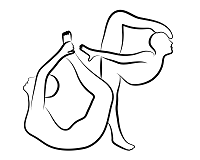OpenPose  1.7.0 The first real-time multi-person system to jointly detect human body, hand, facial, and foot keypoints
op::Point< T > Struct Template Reference

`#include <point.hpp>`

## Public Member Functions

Point (const T x=0, const T y=0)

Point (const Point< T > &point)

Point< T > & operator= (const Point< T > &point)

Point (Point< T > &&point)

Point< T > & operator= (Point< T > &&point)

area () const

std::string toString () const

bool operator< (const Point< T > &point) const

bool operator> (const Point< T > &point) const

bool operator<= (const Point< T > &point) const

bool operator>= (const Point< T > &point) const

bool operator== (const Point< T > &point) const

bool operator!= (const Point< T > &point) const

Point< T > & operator+= (const Point< T > &point)

Point< T > operator+ (const Point< T > &point) const

Point< T > & operator+= (const T value)

Point< T > operator+ (const T value) const

Point< T > & operator-= (const Point< T > &point)

Point< T > operator- (const Point< T > &point) const

Point< T > & operator-= (const T value)

Point< T > operator- (const T value) const

Point< T > & operator*= (const T value)

Point< T > operator* (const T value) const

Point< T > & operator/= (const T value)

Point< T > operator/ (const T value) const

x

y

## Detailed Description

### template<typename T> struct op::Point< T >

Definition at line 10 of file point.hpp.

## ◆ Point() [1/3]

template<typename T >
 op::Point< T >::Point ( const T x = `0`, const T y = `0` )

## ◆ Point() [2/3]

template<typename T >
 op::Point< T >::Point ( const Point< T > & point )

Copy constructor. It performs `fast copy`: For performance purpose, copying a Point<T> or Point<T> or cv::Mat just copies the reference, it still shares the same internal data. Modifying the copied element will modify the original one. Use clone() for a slower but real copy, similarly to cv::Mat and Point<T>.

Parameters
 point Point to be copied.

## ◆ Point() [3/3]

template<typename T >
 op::Point< T >::Point ( Point< T > && point )

Move constructor. It destroys the original Point to be moved.

Parameters
 point Point to be moved.

## ◆ area()

template<typename T >
 T op::Point< T >::area ( ) const
inline

Definition at line 50 of file point.hpp.

## ◆ operator!=()

template<typename T >
 bool op::Point< T >::operator!= ( const Point< T > & point ) const
inline

Not equal comparison operator.

Parameters
 point Point to be compared.
Returns
Whether the instance satisfies the condition with respect to point.

Definition at line 122 of file point.hpp.

## ◆ operator*()

template<typename T >
 Point op::Point< T >::operator* ( const T value ) const

## ◆ operator*=()

template<typename T >
 Point& op::Point< T >::operator*= ( const T value )

## ◆ operator+() [1/2]

template<typename T >
 Point op::Point< T >::operator+ ( const Point< T > & point ) const

## ◆ operator+() [2/2]

template<typename T >
 Point op::Point< T >::operator+ ( const T value ) const

## ◆ operator+=() [1/2]

template<typename T >
 Point& op::Point< T >::operator+= ( const Point< T > & point )

## ◆ operator+=() [2/2]

template<typename T >
 Point& op::Point< T >::operator+= ( const T value )

## ◆ operator-() [1/2]

template<typename T >
 Point op::Point< T >::operator- ( const Point< T > & point ) const

## ◆ operator-() [2/2]

template<typename T >
 Point op::Point< T >::operator- ( const T value ) const

## ◆ operator-=() [1/2]

template<typename T >
 Point& op::Point< T >::operator-= ( const Point< T > & point )

## ◆ operator-=() [2/2]

template<typename T >
 Point& op::Point< T >::operator-= ( const T value )

## ◆ operator/()

template<typename T >
 Point op::Point< T >::operator/ ( const T value ) const

## ◆ operator/=()

template<typename T >
 Point& op::Point< T >::operator/= ( const T value )

## ◆ operator<()

template<typename T >
 bool op::Point< T >::operator< ( const Point< T > & point ) const
inline

Less comparison operator.

Parameters
 point Point to be compared.
Returns
Whether the instance satisfies the condition with respect to point.

Definition at line 72 of file point.hpp.

## ◆ operator<=()

template<typename T >
 bool op::Point< T >::operator<= ( const Point< T > & point ) const
inline

Less or equal comparison operator.

Parameters
 point Point to be compared.
Returns
Whether the instance satisfies the condition with respect to point.

Definition at line 92 of file point.hpp.

## ◆ operator=() [1/2]

template<typename T >
 Point& op::Point< T >::operator= ( const Point< T > & point )

Copy assignment. Similar to Point<T>(const Point<T>& point).

Parameters
 point Point to be copied.
Returns
The resulting Point.

## ◆ operator=() [2/2]

template<typename T >
 Point& op::Point< T >::operator= ( Point< T > && point )

Move assignment. Similar to Point<T>(Point<T>&& point).

Parameters
 point Point to be moved.
Returns
The resulting Point.

## ◆ operator==()

template<typename T >
 bool op::Point< T >::operator== ( const Point< T > & point ) const
inline

Equal comparison operator.

Parameters
 point Point to be compared.
Returns
Whether the instance satisfies the condition with respect to point.

Definition at line 112 of file point.hpp.

## ◆ operator>()

template<typename T >
 bool op::Point< T >::operator> ( const Point< T > & point ) const
inline

Greater comparison operator.

Parameters
 point Point to be compared.
Returns
Whether the instance satisfies the condition with respect to point.

Definition at line 82 of file point.hpp.

## ◆ operator>=()

template<typename T >
 bool op::Point< T >::operator>= ( const Point< T > & point ) const
inline

Greater or equal comparison operator.

Parameters
 point Point to be compared.
Returns
Whether the instance satisfies the condition with respect to point.

Definition at line 102 of file point.hpp.

## ◆ toString()

template<typename T >
 std::string op::Point< T >::toString ( ) const

It returns a string with the whole Point<T> data. Useful for debugging. The format is: `[x, y]`

Returns
A string with the Point<T> values in the above format.

## ◆ x

template<typename T >
 T op::Point< T >::x

Definition at line 12 of file point.hpp.

## ◆ y

template<typename T >
 T op::Point< T >::y

Definition at line 13 of file point.hpp.

The documentation for this struct was generated from the following file: